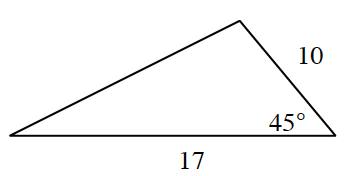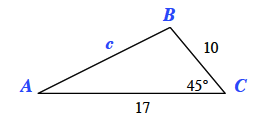### Home > GC > Chapter 5 > Lesson 5.3.5 > Problem5-114

5-114.Solve for the missing side lengths and angles in the triangle at right.

This triangle has known values for $\text{SAS}$ (side-angle-side) so will need to be solved using the Law of Cosines.
Refer to the Math Notes box in Lesson 5.3.3 if you need help remembering the Law of Cosines.Solve for $c$.

$c^2=10^2+17^2-2(10)(17)\text{cos }45º$

After finding the length of $c$, the Law of Sines can be used to to find the measure of $∠A$.
Refer to the Math Notes box in Lesson 5.3.2 for the Law of Sines.

$\frac{\text{sin }A}{10}=\frac{\text{sin }45}{c}$

Use the Triangle Angle Sum Theorem to find the measure of $∠B$.